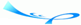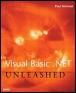# Working with RecursionTeam-FlyVisual Basic .NET Unleashed By Paul Kimmel Table of Contents Chapter 5.  Subroutines, Functions, and Structures

Working with Recursion

Recursion refers to procedures calling themselves . An example of a recursive function was introduced in Listing 5.1; the Factorial function was implemented as a recursive function. Each time Factorial was called, one step of the equation was solved .

Recursive procedures call themselves before exiting until all recursions are complete. Using recursion as shown in the Factorial function can be problematic because this function will be called recursively n - 1 times where n is the initial value of the Value parameter. If Value were very large in the call to Factorial, you could end up running out of memory before all the recursive calls to Factorial could be handled.

Visual Basic .NET allows you to define very large procedural variables ; I was able to declare an array of one hundred million 32-bit integers on my PC with 256MB of RAM.

To compare VB6 to Visual Basic .NET stack memory, I wrote two identical programs. Both programs called a subroutine BlowStack, which recursed infinitely, incrementing a static counter and updating the form's Caption (Text in Visual Basic .NET) property. The VB6 application recursed about 6,000 times before running out of stack space. The Visual Basic .NET application recursed 25,000 times before going into limbo. It appears as though the VB6 application ran out of stack space, and the Visual Basic .NET application just consumed all available memory. Keep in mind, though, that 25,000 anything isn't very much in a computer.

The integrated help doesn't cover the topic of stack memory in any detail, and unlike other languages, the amount of memory allocated to the stack isn't user -configurable. It appears as though Visual Basic .NET just uses whatever memory is available.

#### Defining Recursive Procedures

Another example of recursive procedures is given in the "Quick Sort " section in Chapter 9.

The number of times a quick sort will recur is relatively low. Thus the quick sort works very well using recursion and recursion helps simplify the implementation of the quick sort.

#### Removing Recursion

Recursive algorithms use the procedure as a looping mechanism. Think of the procedure as the loop control mechanism and the implementation of the procedure as the code in the loop. Generally, then, to remove recursion, you replace the call to the recursive procedure with a loop.

Applying this technique to the recursive factorial algorithm, Listing 5.14 demonstrates the Factorial function as a non-recursive function.

##### Listing 5.14 Calculating N -Factorial non-recursively
`  1:  Public Function Factorial2(ByVal Value As Long) As Long  2:  Debug.Assert(Value > 0)  3:  Check(Value)  4:  Dim I As Long  5:  Dim Sum As Long = 1  6:   7:  For I = Value To 2 Step -1  8:  Sum *= I  9:  Next  10:   11:  Return Sum  12:  End Function `

Listing 5.1 called Factorial(Value-1) as long as Value was greater than 1. In Listing 5.14, the upper bound of the For loop represents the Value part of the call to the recursive Factorial algorithm and Step -1 represents subtracting 1 from Value for each recursive call. Looping down to 2 replaces the If (Value > 1) Then test in Listing 5.1. (The Check subroutine implementation is shown in Listing 5.1.) The loop also could have been implemented using a While End While statement:

` While (Value > 1)   Sum *= Value   Value -= 1 End While `

Here we see the loop control as Value > 1, mirroring line 16 of Listing 5.1 exactly, and the decrement of Value as is done in the recursive call on line 17 of Listing 5.1.

Generally, recursion can be removed by using the recursive test and terminate condition as the bounding mechanism for a loop. The test and terminate condition of the recursive factorial algorithm in Listing 5.1 is Value > 1. To create the termination circumstance, Value is decremented after each pass of the recursive call or the loop.

Tip

Recursive algorithms are sometimes easier to implement and often more efficient, but replacing recursive algorithms with loops usually results in more readable code.Team-FlyTopVisual BasicR. NET Unleashed
ISBN: N/A
EAN: N/A
Year: 2001
Pages: 222